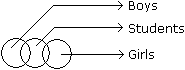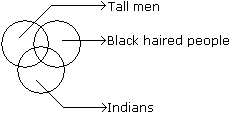Courses

# Test: Venn Diagrams- 2

## 20 Questions MCQ Test Logical Reasoning (LR) and Data Interpretation (DI) | Test: Venn Diagrams- 2

Description
This mock test of Test: Venn Diagrams- 2 for UPSC helps you for every UPSC entrance exam. This contains 20 Multiple Choice Questions for UPSC Test: Venn Diagrams- 2 (mcq) to study with solutions a complete question bank. The solved questions answers in this Test: Venn Diagrams- 2 quiz give you a good mix of easy questions and tough questions. UPSC students definitely take this Test: Venn Diagrams- 2 exercise for a better result in the exam. You can find other Test: Venn Diagrams- 2 extra questions, long questions & short questions for UPSC on EduRev as well by searching above.
QUESTION: 1

### Directions to Solve Each of these questions given below contains three elements. These elements may or may not have some inter linkage. Each group of elements may fit into one of these diagrams at (A), (B), (C), (D) and/or (E). You have to indicate the group of elements which correctly fits into the diagrams. Question - Which of the following diagrams indicates the best relation between Travelers, Train and Bus ?

Solution:

Bus and Train are different from each other but some travelers travel by bus and some travel by train.

QUESTION: 2

### Directions to Solve Each of these questions given below contains three elements. These elements may or may not have some inter linkage. Each group of elements may fit into one of these diagrams at (A), (B), (C), (D) and/or (E). You have to indicate the group of elements which correctly fits into the diagrams. Question - Which of the following diagrams indicates the best relation between Profit, Dividend and Bonus ?

Solution:

Bonus and Dividend are different from each other. But both these are parts of profit.

QUESTION: 3

### Directions to Solve Each of these questions given below contains three elements. These elements may or may not have some inter linkage. Each group of elements may fit into one of these diagrams at (A), (B), (C), (D) and/or (E). You have to indicate the group of elements which correctly fits into the diagrams. Question - Which of the following diagrams indicates the best relation between Women, Mothers and Engineers ?

Solution:

All mothers are women and some mothers and some women may be engineers.

QUESTION: 4

Directions to Solve

Each of these questions given below contains three elements. These elements may or may not have some inter linkage. Each group of elements may fit into one of these diagrams at (A), (B), (C), (D) and/or (E). You have to indicate the group of elements which correctly fits into the diagrams.

Question -

Which of the following diagrams indicates the best relation between Author, Lawyer and Singer ?

Solution:

All the three are different professions.

QUESTION: 5

Directions to Solve

Each of these questions given below contains three elements. These elements may or may not have some inter linkage. Each group of elements may fit into one of these diagrams at (A), (B), (C), (D) and/or (E). You have to indicate the group of elements which correctly fits into the diagrams.

Question -

Which of the following diagrams indicates the best relation between Factory, Product and Machinery ?

Solution:

Product and Machinery are different from each other but both are found in Factory.

QUESTION: 6

Directions to Solve

Each of these questions given below contains three elements. These elements may or may not have some inter linkage. Each group of elements may fit into one of these diagrams at (A), (B), (C), (D) and/or (E). You have to indicate the group of elements which correctly fits into the diagrams.

Question -

Which of the following diagrams indicates the best relation between Judge, Thieves and Criminals ?

Solution:

All the thieves are criminals while judge is different from these.

QUESTION: 7

Directions to Solve

Each of these questions given below contains three elements. These elements may or may not have some inter linkage. Each group of elements may fit into one of these diagrams at (A), (B), (C), (D) and/or (E). You have to indicate the group of elements which correctly fits into the diagrams.

Question -

Which of the following diagrams indicates the best relation between Pigeon, Bird and Dog ?

Solution:

All the pigeons are birds while dog is different from these.

QUESTION: 8

Directions to Solve

Each of these questions given below contains three elements. These elements may or may not have some inter linkage. Each group of elements may fit into one of these diagrams at (A), (B), (C), (D) and/or (E). You have to indicate the group of elements which correctly fits into the diagrams.

Question -

Which of the following diagrams indicates the best relation between India, Haryana and World ?

Solution:

Haryana is in India and India is in the World.

QUESTION: 9

Directions to Solve

Each of these questions given below contains three elements. These elements may or may not have some inter linkage. Each group of elements may fit into one of these diagrams at (A), (B), (C), (D) and/or (E). You have to indicate the group of elements which correctly fits into the diagrams.

Question -

Which of the following diagrams indicates the best relation between Earth, Sea and Sun ?

Solution:

Sea is a part of Earth while Sun is different from these two.

QUESTION: 10

Directions to Solve

Each of these questions given below contains three elements. These elements may or may not have some inter linkage. Each group of elements may fit into one of these diagrams at (A), (B), (C), (D) and/or (E). You have to indicate the group of elements which correctly fits into the diagrams.

Question -

Which of the following diagrams indicates the best relation between Hockey, Football and Cricket ?

Solution:

All these three games are different from each other.

QUESTION: 11

Directions to Solve

Each of these questions given below contains three elements. These elements may or may not have some inter linkage. Each group of elements may fit into one of these diagrams at (A), (B), (C), (D) and/or (E). You have to indicate the group of elements which correctly fits into the diagrams.

Question -

Which of the following diagrams indicates the best relation between Iron, Lead and Nitrogen ?

Solution:

All these three elements are different from each other

QUESTION: 12

Directions to Solve

Each of these questions given below contains three elements. These elements may or may not have some inter linkage. Each group of elements may fit into one of these diagrams at (A), (B), (C), (D) and/or (E). You have to indicate the group of elements which correctly fits into the diagrams.

Question -

Which of the following diagrams indicates the best relation between Examination, Questions and Practice ?

Solution:

Some questions are asked in examination and some in practice but examination and practice are different from each other.

QUESTION: 13

Directions to Solve

Each of these questions given below contains three elements. These elements may or may not have some inter linkage. Each group of elements may fit into one of these diagrams at (A), (B), (C), (D) and/or (E). You have to indicate the group of elements which correctly fits into the diagrams.

Question -

Which of the following diagrams indicates the best relation between Bulb, Lamp and Light ?

Solution:

Bulb and Lamp are different from each other but light is obtained from both.

QUESTION: 14

Directions to Solve

Each of these questions given below contains three elements. These elements may or may not have some inter linkage. Each group of elements may fit into one of these diagrams at (A), (B), (C), (D) and/or (E). You have to indicate the group of elements which correctly fits into the diagrams.

Question -

Which of the following diagrams indicates the best relation between Moon, Sun and Earth ?

Solution:

All the three are different from each other.

QUESTION: 15

Directions to Solve

Each of these questions given below contains three elements. These elements may or may not have some inter linkage. Each group of elements may fit into one of these diagrams at (A), (B), (C), (D) and/or (E). You have to indicate the group of elements which correctly fits into the diagrams.

Question -

Which of the following diagrams indicates the best relation between Lion, Dog and Snake ?

Solution:

All the three are different from each other.

QUESTION: 16

Directions to Solve

Each of these questions given below contains three elements. These elements may or may not have some inter linkage. Each group of elements may fit into one of these diagrams at (A), (B), (C), (D) and/or (E). You have to indicate the group of elements which correctly fits into the diagrams.

Question -

Which of the following diagrams indicates the best relation between Hospital, Nurse and Patient ?

Solution:

Hospital consists of nurse and patient but nurse and patient are of two different nature.

QUESTION: 17

Directions to Solve

Each of these questions given below contains three elements. These elements may or may not have some inter linkage. Each group of elements may fit into one of these diagrams at (A), (B), (C), (D) and/or (E). You have to indicate the group of elements which correctly fits into the diagrams.

Question -

Which of the following diagrams indicates the best relation between Mercury, Zinc and Metal ?

Solution:

Mercury and Zinc both are different from one another but belong to metal.

QUESTION: 18

Directions to Solve

Each of these questions given below contains three elements. These elements may or may not have some inter linkage. Each group of elements may fit into one of these diagrams at (A), (B), (C), (D) and/or (E). You have to indicate the group of elements which correctly fits into the diagrams.

Question -

Which of the following diagrams indicates the best relation between Teacher, Writer and Musician ?

Solution:

A teacher may or may not be a writer and musician. Similarly a musician may or may not be a teacher and writer and so a writer may or may not be a teacher and musician.

QUESTION: 19

Directions to Solve

Each of these questions given below contains three elements. These elements may or may not have some inter linkage. Each group of elements may fit into one of these diagrams at (A), (B), (C), (D) and/or (E). You have to indicate the group of elements which correctly fits into the diagrams.

Question -

Which of the following diagrams indicates the best relation between Boys, Girls and Students ?

Solution:Two items are related to a third item to some extent but not completely and first two items totally different. (Students, boys, girls)

The boys and girls are different items while some boys may be students.

QUESTION: 20

Directions to Solve

Each of these questions given below contains three elements. These elements may or may not have some inter linkage. Each group of elements may fit into one of these diagrams at (A), (B), (C), (D) and/or (E). You have to indicate the group of elements which correctly fits into the diagrams.

Question -

Which of the following diagrams indicates the best relation between Tall man, Black haired people and Indians ?

Solution: